#### CovariantD

##### Description

CovariantD[mu, a, b] is the covariant derivative for a bosonic field. CovariantD[mu] is the covariant derivative for a fermionic field. CovariantD[OPEDelta, a, b] is a short form for CovariantD[mu,a,b]*FourVector[OPEDelta, mu]. CovariantD[{OPEDelta, a, b}, {n}] yields the product of m operators., where n is an integer. CovariantD[OPEDelta, a, b, {m, n}] gives the expanded form of CovariantD[OPEDelta, a, b]^m up to order g^n for the gluon, where n is an integer and g the couplingconstant indicated by the setting of the option CouplingConstant. CovariantD[OPEDelta, {m, n}] gives the expanded form of CovariantD[OPEDelta]^m up to order g^n of the fermionic field.

``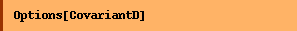``
`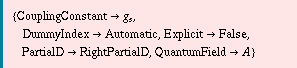`

Possible settings of PartialD are: LeftPartialD, LeftRigthPartialD, RightPartialD. The default setting of QuantumField is GaugeField.

##### Examples
``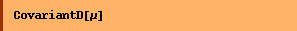``
`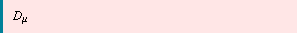`
``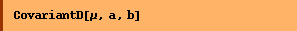``
`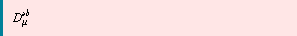`
``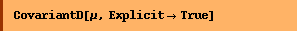``
`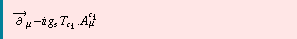`

The first argument of CovariantD is intepreted as type LorentzIndex, except for OPEDelta, which is type Momentum.

``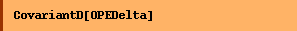``
`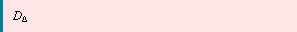`
``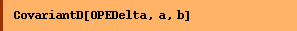``
`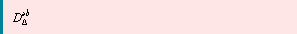`
``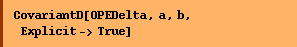``
`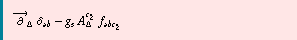`
``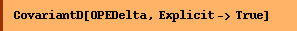``
`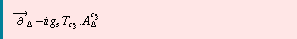`
``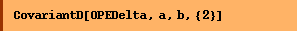``
`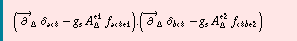`

This gives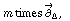the partial derivativecontracted with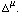``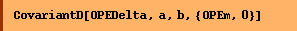``
`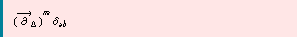`

The expansion up to first order in the coupling constant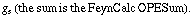``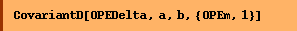``
`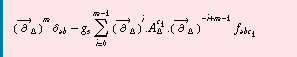`

The expansion up to second order in the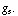``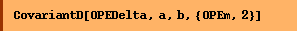``
`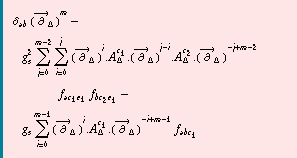`
``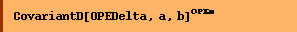``
`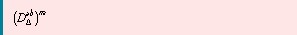`
``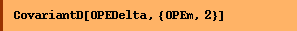``
`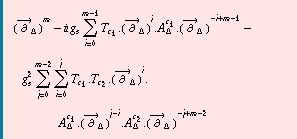`
``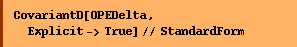``
`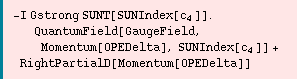`
``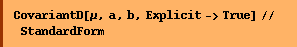``
`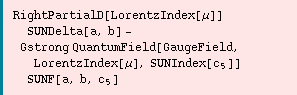`

Converted from the Mathematica notebook CovariantD.nb